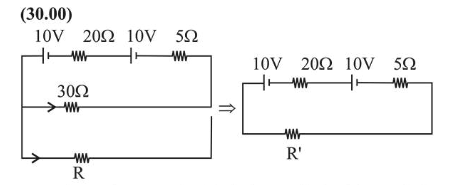# The series combination of two batteries,Question:

The series combination of two batteries, both of the same emf $10 \mathrm{~V}$, but different internal resistance of $20 \Omega$ and $5 \Omega$, is connected to the parallel combination of two resistors $30 \Omega$ and $\mathrm{R} \Omega$. The voltage difference across the battery of internal resistance $20 \Omega$ is zero, the value of $R$ (in $\Omega$ ) is _______

Solution:The resistance of $30 \Omega$ is in parallel with $R$. Their effective resistance

$\frac{1}{R^{\prime}}=\frac{1}{30}+\frac{1}{R}$

$R^{\prime}=\frac{30 R}{30+R}$                        ....(1)

Also, V $=\mathrm{IR}$

$\Rightarrow 10=\frac{20 \times 20}{R^{\prime}+25}$

$\Rightarrow R^{\prime}+25=40$

$\Rightarrow R^{\prime}=15$

$R^{\prime}=15=\frac{30 R}{30+R}$              Using (i)

$\Rightarrow 30+R=2 R$

$\Rightarrow R=30 \Omega$